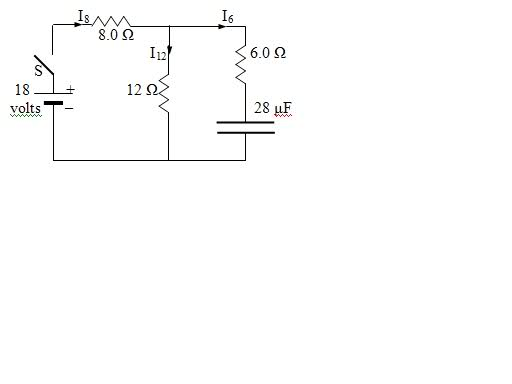# Charge on capacitor (RC circuit)

cashmoney805

## Homework Statement

1) Determine the current in each resistor and charge on the capacitor after a long time immediately after the switch is closed
2) Determine the current in each resistor and charge on the capacitor after a long time

After the switch has been closed for a long time it is re-opened.

3) Determine the current in each resistor and the charge on the capacitor immediately after the switch has been re-opened.
4) Determine the time constant of the capacitor discharge.V = I Req
Q = CV

## The Attempt at a Solution

I got #1 and got I6 = 1A, I8 = 1.5A, and I12 = .5A
I'm not sure what to do about #2 though. What is the voltage across the capacitor? I thought it would be 6V, because that is the voltage across the parallel series. However, there is a voltage drop after the 1A current runs across the 6ohm resistor so the V across the capacitor is 0.

For #3 and 4, does the 8ohm resistor come into play or no?
Thanks for the help guys.

Last edited:

cashmoney805
Is the V across the capacitor just 6V? If so I think I'm good. #2 I through the 6ohm resistor is 0, then I through the other two resistors is 18V/20ohms right?
#3 I = 6V/18ohms Q= 28 E-6 * 6
#4 (18 * 28E-6) = RC

Homework Helper
Voltage which charges the capacitor is the voltage across 12 ohm resistance. Calculate that voltage.
When the capacitor is completely charged, the same voltage will be the voltage across it.

cashmoney805
oh is this Kirchoff's rule?

Homework Helper
No. There is no need to apply Kirchhoff rule.